The power dissipated in the circuit shown in

the figure is 30 Watt. The value of R is :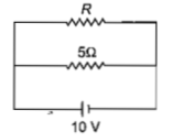(a) $20\mathrm{\Omega }$

(b) $15\mathrm{\Omega }$

(c) $10\mathrm{\Omega }$

(d) $30\mathrm{\Omega }$

Concept Questions :-

Heating effect of current
High Yielding Test Series + Question Bank - NEET 2020

Difficulty Level:

A cell having an emf $\mathrm{\epsilon }$ internal resistance r

is connected across a variable external

resistance R. As the resistance R is increased,

the plot of potential difference V across R is given

by

(a)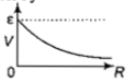(b)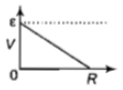(c)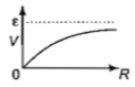(d)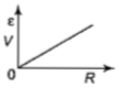Concept Questions :-

Emf and terminal voltage
High Yielding Test Series + Question Bank - NEET 2020

Difficulty Level:

If power dissipated in the 9$\mathrm{\Omega }$ resistor in the circuit

shown is 36 W, the potential difference across

the 2$\mathrm{\Omega }$ resistor is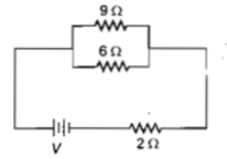(a) 8 V

(b) 10 V

(c) 2 V

(d) 4 V

Concept Questions :-

Heating effect of current
High Yielding Test Series + Question Bank - NEET 2020

Difficulty Level:

A current of 2A flows through a 2$\mathrm{\Omega }$ resistor

when connected across a battery. The same

battery supplies a current of 0.5 A when

connected across a 9$\mathrm{\Omega }$ resistor. The internal

resistance of the battery is

(a) $1/3\mathrm{\Omega }$                   (b) $1/4\mathrm{\Omega }$

(c) $1\mathrm{\Omega }$                       (d) $0.5\mathrm{\Omega }$

Concept Questions :-

Emf and terminal voltage
High Yielding Test Series + Question Bank - NEET 2020

Difficulty Level:

In the circuit in the figure, if the potential at point A is taken to be zero, the potential at point B is :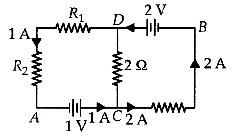1. -1V                                          2. +2V

3. -2V                                          4. +1V

Concept Questions :-

Kirchoff's voltage law
High Yielding Test Series + Question Bank - NEET 2020

Difficulty Level:

Which one of the following bonds produces a solid that reflects light in the visible region and whose electrical conductivity decreases with temperature and has high melting point?

(a) metallic bonding

(b) van der Waals'bonding

(c) ionic bonding

(d) covalent bonding

Concept Questions :-

Current and current density
High Yielding Test Series + Question Bank - NEET 2020

Difficulty Level:

A potentiometer circuit is set up as shown.The potential gradient across the potentiometer wire, is k volt/cm and the ammeter, present in the circuit, reads 1.0A when two way key is switched off. The balance points, when the key between the terminals (i) 1 and 2 (ii) 1and 3, is plugged in, are found to be at lengths  respectively.The magnitudes, of the resistors R and X, in ohm, are then, equal, respectively, to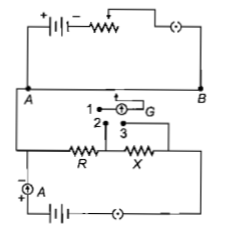(a)

(b)

(c)

(d)

Concept Questions :-

Meter bridge and potentiometer
High Yielding Test Series + Question Bank - NEET 2020

Difficulty Level:

Consider the following two statements :

(A) Kirchhoff's junction law follows from the conservation of charge.

(B) Kirchhoff's loop law follows from the conservation of energy.

Which of the following is correct?

(a) Both (A) and (B) are wrong

(b) (A) is correct and (B) is wrong

(c) (A)  is wrong and (B) is correct

(d) Both (A) and (B) are correct

Concept Questions :-

Kirchoff's voltage law
High Yielding Test Series + Question Bank - NEET 2020

Difficulty Level:

A wire of resistance  is bent to form a complete circle of radius 10 cm. The resistance between its two diametrically opposite points, A and B as shown in the figure, is :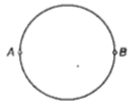(a)                                                (b)

(c)                                                   (d)

Concept Questions :-

Combination of resistors
High Yielding Test Series + Question Bank - NEET 2020

Difficulty Level:

A student measures the terminal potential difference (V) of a cell (of emf $\epsilon$ and internal resistance r) as a function of the current (I) flowing through it. The slope and intercept of the graph between V and I, then respectively, equal :

(a)                                (b)

(c)                                (d)

Concept Questions :-

Emf and terminal voltage